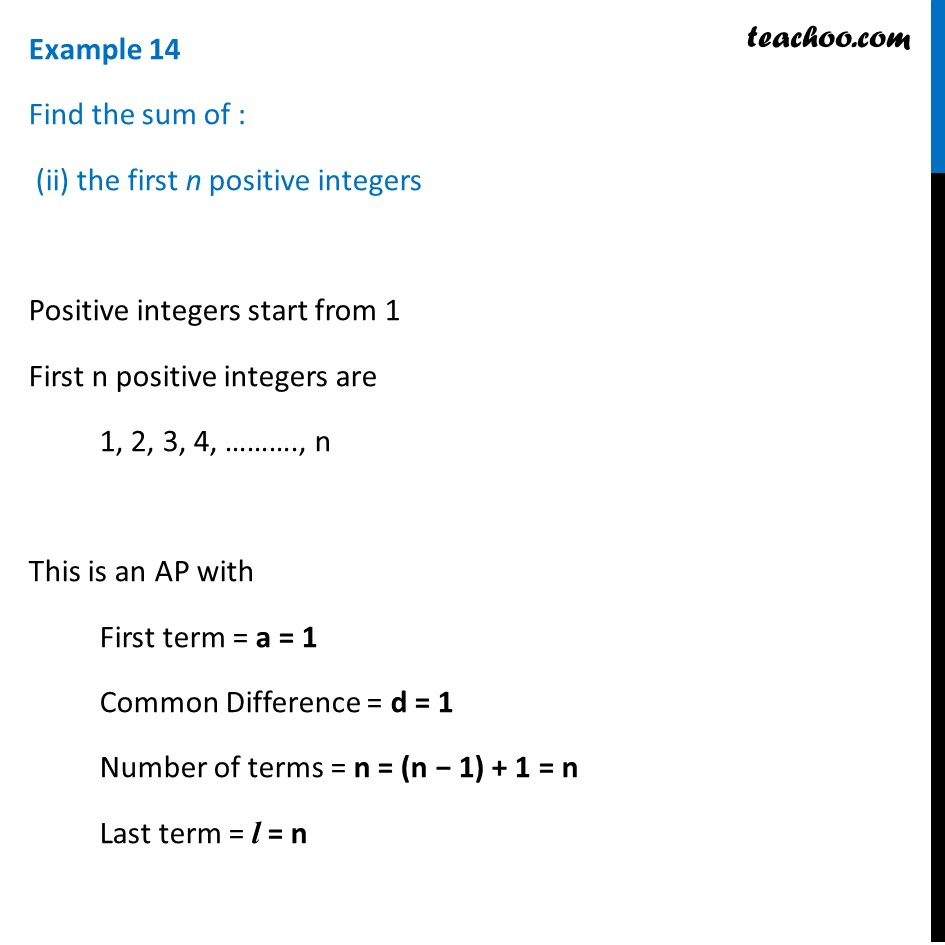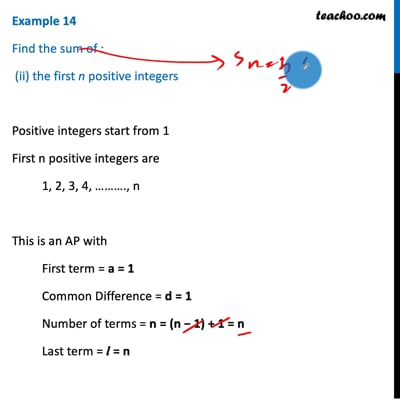Examples

Chapter 5 Class 10 Arithmetic Progressions
Serial order wiseThis video is only available for Teachoo black users

Introducing your new favourite teacher - Teachoo Black, at only ₹83 per month

### Transcript

Example 14 Find the sum of : (ii) the first n positive integers Positive integers start from 1 First n positive integers are 1, 2, 3, 4, ………., n This is an AP with First term = a = 1 Common Difference = d = 1 Number of terms = n = (n − 1) + 1 = n Last term = l = n To find sum, we use the formula Sum = 𝒏/𝟐[𝒂+𝒍] Putting values Sum = 𝑛/2 (1+𝑛) Sum = (𝑛(𝒏 + 𝟏))/2